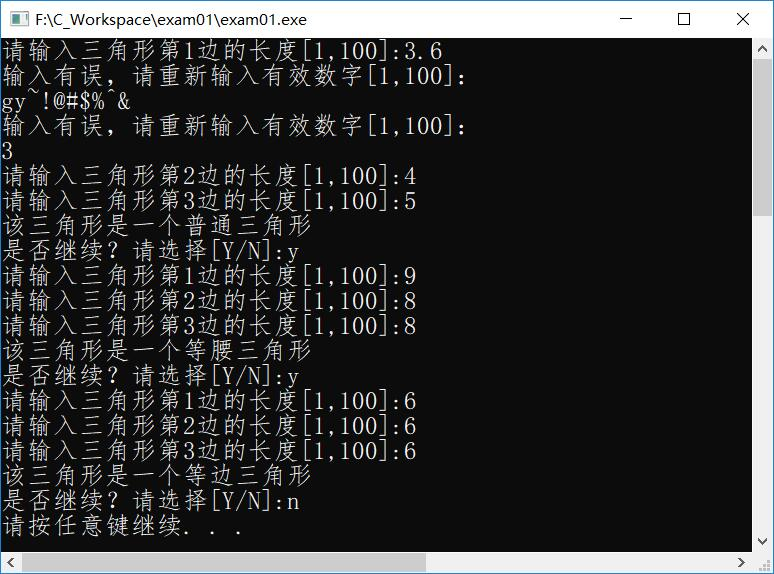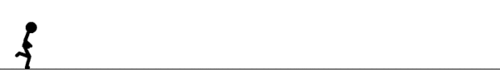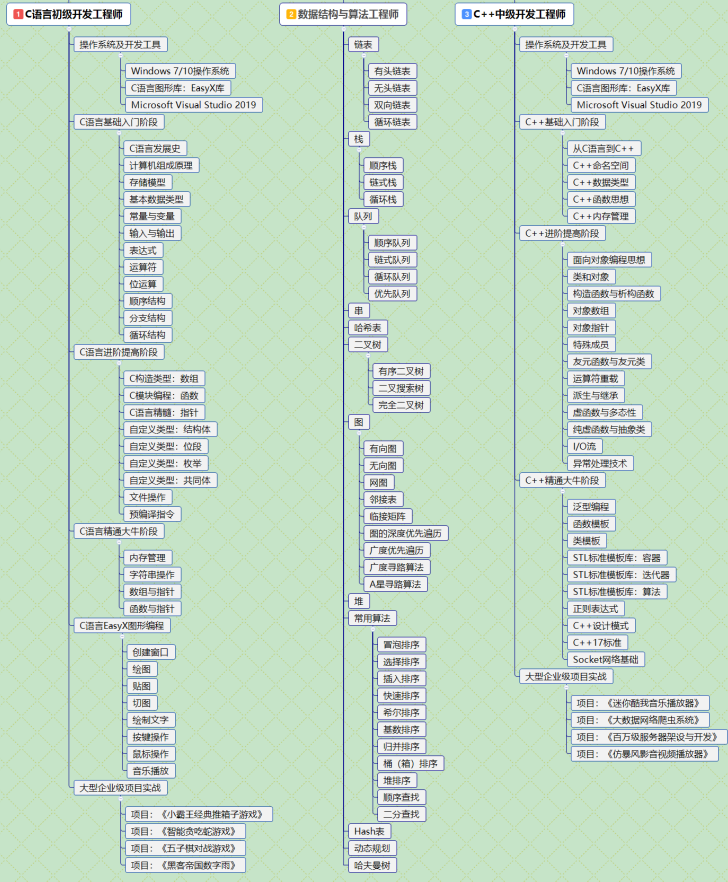•Java
• java中判断是否三角形的方法：package Angle;import java.util.Scanner;public class Angle {//判断是否能构成三角形public static int JudgeAngle(int a,int b,int c){if(a+b>c&&a+c>b&&b+c&...
java中判断是否三角形的方法：package Angle;import java.util.Scanner;public class Angle {//判断是否能构成三角形public static int JudgeAngle(int a,int b,int c){if(a+b>c&&a+c>b&&b+c>a){System.out.println("能够构成三角形！");return 1;}else{System.out.println("不能构成三角形!");return 0;}}//判断是否能构成等腰三角形public static int JudgeDangle(int a,int b,int c){if(a==b||a==c||b==c){if(a==b&&a==c){System.out.println("该三角形是等边三角形！");return 1;}else{System.out.println("该三角形是普通的等腰三角形！");return 1;}}else{return 0;}}//判断是否是直角三角形public static int JudgeRangle(int a,int b,int c){int r1,r2,r3;r1=a*a+b*b-c*c;r2=a*a+c*c-b;r3=b*b+c*c-a*a;/*System.out.println(r1+r2+r3);*/if(r1==0||r2==0||r3==0){System.out.println("该三角形是直角三角形！");return 1;}else{//System.exit(0);return 0;}}public static void main(String [] args){int a;int b;int c;System.out.println("请输入三角形的三边: ");Scanner scanner=new Scanner(System.in);a=scanner.nextInt();b=scanner.nextInt();c=scanner.nextInt();//判断输入三边是否合法if(a<0||a>200||b<0||b>200||c<0||c>200){System.out.println("你输入的三边不合法！");}else{//判断是否能构成三角形JudgeAngle(a,b,c);//判断是否是等腰或等边三角形JudgeDangle(a,b,c);//判断是否是直角三角形JudgeRangle(a,b,c);//判断是一般三角形System.out.println("该三角形是一般三角形!");}}}上述代码通过判断三边的长度来判断是否三角形。
展开全文• 2.三角形的三边长度为整数且限制在1~100之间。 3.有良好的排错机制。（程序不接受非数字字符等） 4.友好的交互提示。（输入错误提示，是否继续提示等） 【开发环境】 1.windows10 2.Dev-C++ 5.1.0 【程序源码...
【需求描述】

1.使用C++语言编写程序。

2.三角形的三边长度为整数且限制在1~100之间。

3.有良好的排错机制。（程序不接受非数字字符等）

4.友好的交互提示。（输入错误提示，是否继续提示等）

【开发环境】

1.windows10

2.Dev-C++ 5.1.0

【程序源码】

#include <iostream>
#include <limits>
#include <sstream>

using namespace std;

class Triangle{
private:
int length_a,length_b,length_c;
public:
Triangle(int a,int b,int c){
length_a=a;
length_b=b;
length_c=c;
}
~Triangle(){
}

//测试单边长度是否在要求范围
bool lengthtest(int tlen) {
if(tlen>=1&&tlen<=100){
return true;
}else{
return false;
}
}

/*校验三边长度是否合法的方法*/
bool lengthCheck(){
/*判断三边的值是否在要求的范围*/
if(lengthtest(length_a)){
if(lengthtest(length_b)){
if(lengthtest(length_c)){
return true;
}
}
}
return false;
}

//	校验三边长度是否可以组成三角形
bool composeCheck(){
if(length_a+length_b>length_c){
if(length_b+length_c>length_a){
if(length_a+length_c>length_b){
return true;
}
}
}
return false;
}

//判断三角形的类型
int TypeCheck(){
if(length_a==length_b||length_a==length_c||length_b==length_c){
if(length_a==length_b&&length_b==length_c){
return 3;
}
return 2;
}
return 1;
}

};
int main() {

int a,b,c;
if(!isdigit(a)){
}
A:for(int i=1;i<=3;i++) {
cout<<"请输入三角形第"<<i<<"边的长度[1,100]:";
int num;
while( !(cin>>num) || cin.peek()!='\n' )
{
cin.clear();
cin.ignore( numeric_limits<streamsize>::max(), '\n' );
cout << "输入有误，请重新输入有效数字[1,100]：" << endl;
}
if(i==1){
a=num;
}else if(i==2){
b=num;
}else{
c=num;
}
}
Triangle triangle(a,b,c);
if(!triangle.lengthCheck()){
cout<<"输入的三边长度超出范围,请重新输入:"<<endl;
triangle.~Triangle();
goto A;
}

if(triangle.composeCheck())	{
int temp = triangle.TypeCheck();
switch(temp)
{
case 1:cout<<"该三角形是一个普通三角形"<<endl;break;
case 2:cout<<"该三角形是一个等腰三角形"<<endl;break;
case 3:cout<<"该三角形是一个等边三角形"<<endl;break;
default:break;
}
}else{
cout<<"输入的三边无法组成三角形。"<<endl;
}
cout<<"是否继续？请选择[Y/N]:";
char d;
cin>>d;
if(d==89||d==121) {
triangle.~Triangle();
goto A;
}
system("pause");
return 0;
}

【运行测试效果】【改进意见】

本次实验由于受到需求束缚，无法拓展更多功能，正常情况下，可以有以下改进：

1.三角形类型判断应该可以更加丰富：普通、等腰锐角三角形，普通、等腰钝角三角形，普通、等腰直角三角形。

2.支持输入小数作为边长。

3.不强制性三边数据都要输入，但有提示输入数据不完整以及重输措施。

展开全文• 根据输入的三角形的三条边判断三角形的类型，并输出其面积和类型。 算法思想 首先判断其两边之和是否大于第三边，若大于则判断可以构成三角形，再进一步判断该三角形是什么三角形，并计算这个三角形的面积；否则...
根据输入的三角形的三条边判断三角形的类型，并输出其面积和类型。

算法思想

首先判断其两边之和是否大于第三边，若大于则判断可以构成三角形，再进一步判断该三角形是什么三角形，并计算这个三角形的面积；否则不能构成三角形。

① 从键盘输入三角形的三条边。

② 判断两边之和是否大于第三边。

③ 若条件成立则判断可构成三角形，计算其面积，并判断其类型；否则判断其不能构成三角形。

④ 在类型判断中首先判断其是否三边相等，条件成立则判断其为等边三角形；否则判断其是否有两边相等，条件成立则判断其为等腰三角形；否则判断其是否有两边的平方和等于第三边的平方，条件成立则判断其为直角三角形；否则判断其为普通三角形。

程序代码

#include <stdio.h>

#include <math.h>

int main()

{

float a,b,c;

float s,area;

printf("请输入三角形的三条边:\n");

scanf("%f,%f,%f",&a,&b,&c);

if(a+b>c&&b+c>a&&a+c>b)

{

s=(a+b+c)/2;

area=(float)sqrt(s*(s-a)*(s-b)*(s-c));

printf("三角形的面积是：%f\n" ,area);

if(a==b&&a==c)

printf("三角形是等边三角形\n");

else if(a==b||a==c||b==c)

printf("三角形是等腰三角形\n");

else if((a*a+b*b==c*c)||(a*a+c*c==b*b)||(b*b+c*c==a*a))

printf("三角形是直角三角形\n");

else

printf("三角形是普通三角形\n");

}

else

printf("不能构成三角形\n");

return 0;

}

调试运行结果

输入为 3，3，3 时，对应的输出情况如下所示：

请输入三角形的三条边:

3,3,3

三角形的面积是：3.897114

三角形是等边三角形

输入为 3，4，5 时，对应的输出情况如下所示：

请输入三角形的三条边:

3,4,5

三角形的面积是：6.000000

三角形是直角三角形

总结

① 在本实训之前必须知道三角形的相关知识，比如，怎么判断输入的三边是否能构成三角形、三角形的面积计算方法等。

② 要注意逻辑运算符“&&”和“||”的使用，当需要同时满足多个条件时，使用“&&”运算符；而当只需满足多个条件中的一个时，使用“||”运算符。不管你是转行也好，初学也罢，进阶也可，如果你想学编程，进阶程序员~

【值得关注】我的 编程学习交流俱乐部！【点击进入】展开全文c语言 编程学习 算法 C语言入门
• c语言中有很多有趣程序，以下是百分网小编分享给大家如何判断三角形类型c语言小程序，欢迎阅读!#include#include#define EPSINON 1e-3#define ABS(a) (((a)>0)?(a):(-a)) //?：不支持表达式嵌套#define ...
引导语;c语言中有很多有趣的小程序，以下是百分网小编分享给大家的如何判断三角形类型的c语言小程序，欢迎阅读!#include#include#define EPSINON 1e-3#define ABS(a) (((a)>0)?(a):(-a)) //?：不支持表达式嵌套#define ZERO(x) ((x)>-EPSINON && (x)#define MAX(a,b) (((a)>(b))?(a):(b))#define MIN(a,b) (((a)float a, b, c;float max, mid, min;char input_err_flag = 0;char judge_err_flag = 0;int equal(float a, float b){float tmp;tmp = a - b;tmp = ZERO(ABS(tmp));return tmp;}void input(void){a = b = c = 0;printf("输入三条边的值：");scanf("%f %f %f",&a, &b, &c);if(!(a>0) || !(b>0) || !(c>0)){input_err_flag = 1;}}void sort(void){max = MAX(MAX(a,b),c);min = MIN(MIN(a,b),c);if(MAX(a,b) < c)mid = MAX(a,b);elsemid = MAX(MIN(a,b),c);}void judge(void){float max_square, mid_square, min_square, tmp;if(max >= (mid+min)){judge_err_flag = 1;}else{max_square = max * max;mid_square = mid * mid;min_square = min * min;tmp = mid_square + min_square;if(equal(mid,min) || equal(max, mid)){if(equal(mid, min)){if(mid == max)puts("等边三角形。");else if(equal(max_square, tmp))puts("等腰直角三角形。");else if(max_square < tmp)puts("等腰锐角三角形。");elseputs("等腰钝角三角形。");}else{if(equal(min, mid))puts("等边三角形。");elseputs("等腰锐角三角形。");}}else if(equal(max_square, tmp))puts("直角三角形。");else if(max_square < tmp)puts("锐角三角形。");elseputs("钝角三角形。");}}int main(void){char cs, ch;do{input();sort();judge();if(input_err_flag){input_err_flag = 0;while((cs=getchar())!='n' && (cs=getchar())!=EOF);printf("输入错误，a b c必须大于零，是否新输入(y/n)：");}else if(judge_err_flag){judge_err_flag = 0;while((cs=getchar())!='n' && (cs=getchar())!=EOF);printf("组不成三角形,是否重新输入(y/n)：");}else{while((cs=getchar())!='n' && (cs=getchar())!=EOF);printf("是否再输入一组数据(y/n)：");}ch = getchar();}while(ch=='y' || ch=='Y' || ch=='n');puts("Goodbye!");return 0;}
展开全文• 昨天写了一个判断三角形形状的程序，然后EZ发现了里面的无数个问题。 原本我的程序是 #include int main() { int a,b,c; scanf("%d,%d,%d",&a,&b,&c); if(a+b>c&&b+c>a&&a+c>b) { if(a==b) if(b==c) printf...c
• 三角形构成算法 两边之和大于第三边 ，两边之差小于第三边。 import java.util.Scanner; public class jiejie {public static void main(String[] args) { // TODO Auto-generated method stub int a,b,c;java
• 很简单的程序，而且容易理解和模仿。判断一个三角形是不是等边或等腰的三角形
• 输入三角形的三条边长，判断是否能构成一个三角形（不考虑退化三角形，即面积为零的三角形），是什么样的三角形（直角、锐角、钝角、等边、等腰）。 函数声明为：byte GetTriangleType(int,int,int) 　1. 如何用一个...max函数
• (3）如果能构成三角形，判断三角形是何种三角形。如果三角形的任意一条边的平方等于其他两条边平方的和，则为直角三角形;如果任意一条边的平方大于其他两条边平方的和，则为钝角三角形:否则，为锐角三角形。 输出...
• 根据输入的三角形的三条边判断三角形的类型，并输出他的面积和类型 思想简述 首先分析三角形的类型 三角形等腰三角形直角三角形普通三角形等边三角形等腰直角三角形 看程序框图分支部分要用if语句来操作 求面积要...c语言
• 题目描述 娄月的妹妹刘月是个初中生，某天回来兴高采烈的回家告诉姐姐他学会了如何凭借三角形三边判断...以下是娄月编写的程序，由于忘记了一些知识点，三个判断三角形的函数无法完成，于是 只能请聪明的你帮忙喽。#i
• 1 2 3 4 5 6 7 8 9 10 11 12 13 14 15 16 17 18 19 20 21 22 23 24 25 26 27 28 29 /*please write a program to check three number if can construct a tryaigle*/ #include <i...
• 判断三角形的形状和是否能构成三角形 输入三条边 这个程序属于C# 控制台应用程序
• PAGE / NUMPAGES 题目编写程序读入三角形的三条边并确定输入是否有效如果任意两条边的和大于第三条边则输入有效例如如果输入的三条边是1,2,1输出应该是 1,2,1能否形成三角形false 如果输入的三条边是2,2,1输出应该是...
• // ...// A05 rights reserved. // 作 者：A05黄婉菲 // 完成日期：2014年 11 月1日 // 版 本 号：v1.0 ...// 问题描述：判断三角形的性质 // 输入描述：三个数 // 程序输出：三角形的性质 // using System;
• import java.util.*;class Hu{ int a; int b; int c; void judge() { Scanner in = new Scanner(System.... System.out.println("请输入三角形的三边a，b，c。"); a = in.nextInt(); b = in.nextInt(); c = i测试 class c import string
• #Copyright (c)2017, 东北大学软件学院学生# All rightsreserved#文件名称：a.py# 作 者：孔云#问题描述：编写程序，从键盘输入三条边，判断是否能够构成一个三角形。#问题分析：组成三角形的条件是任意两边之和大于...
• 思路：根据三角形的性质来判读类型（简单） #include using namespace std; int main() { int a,b,c; cin>>a>>b>>c; //三角形的三条边； if(a+b cout else if(a==b&&a==c) //三边相等为等边system c
• # 以下是判断输入值是否为字符，不是字符再继续执行，是字符则结束程序不再执行 if A.isdigit() and B.isdigit() and C.isdigit(): # 'str.isdigit()'判断某个字符串是数字还是非数字' A = float(A) B = float(B)......